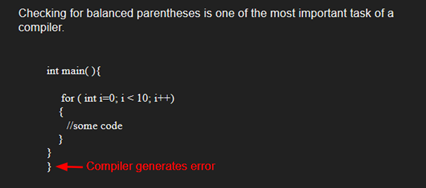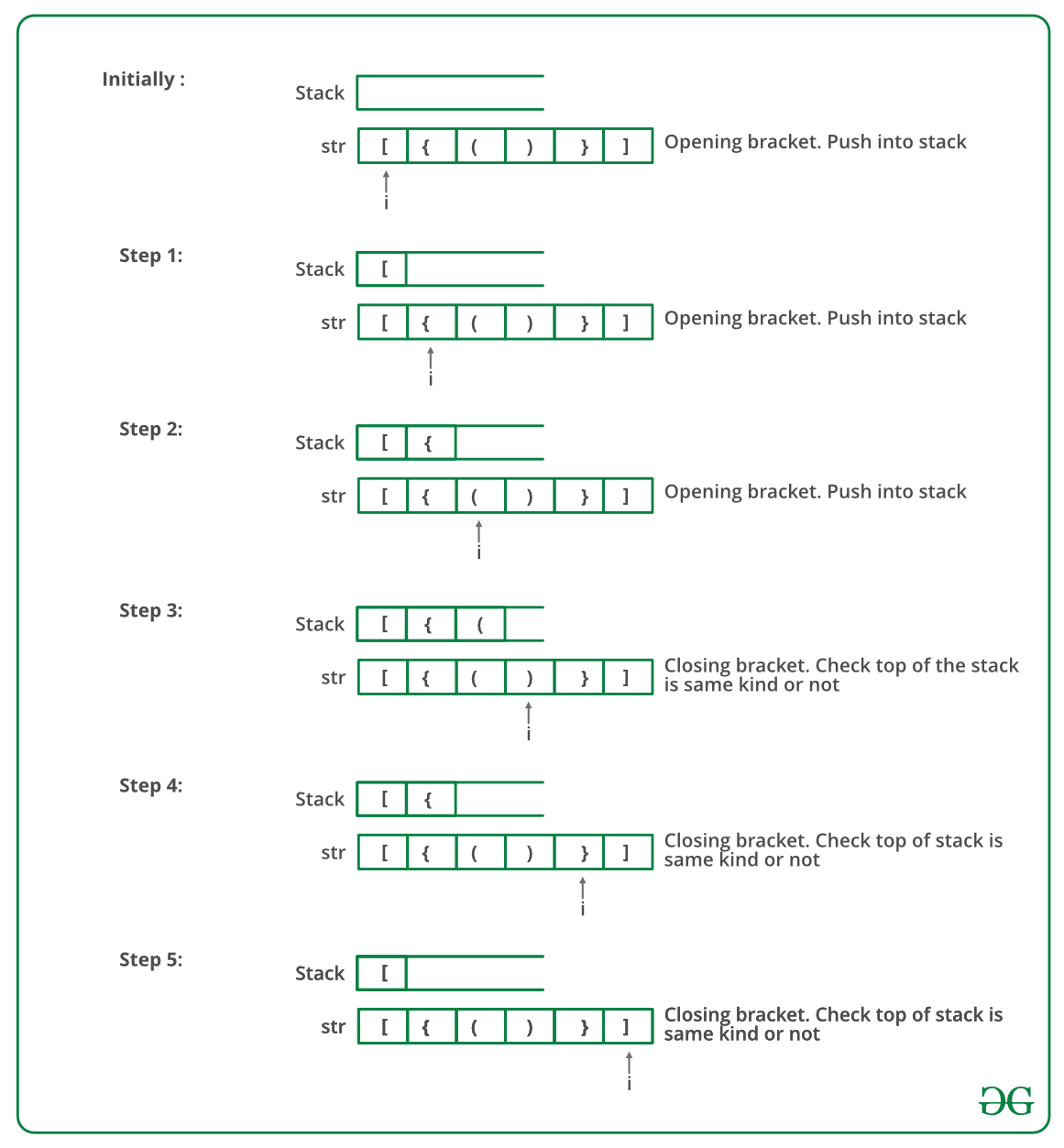GFG App
Open AppBrowser
Continue

# C Program To Check For Balanced Brackets In An Expression (Well-Formedness) Using Stack

Given an expression string exp, write a program to examine whether the pairs and the orders of “{“, “}”, “(“, “)”, “[“, “]” are correct in exp.

Example

Input: exp = “[()]{}{[()()]()}”
Output: Balanced

Input: exp = “[(])”
Output: Not BalancedAlgorithm:

• Declare a character stack S.
• Now traverse the expression string exp.
1. If the current character is a starting bracket (‘(‘ or ‘{‘ or ‘[‘) then push it to stack.
2. If the current character is a closing bracket (‘)’ or ‘}’ or ‘]’) then pop from stack and if the popped character is the matching starting bracket then fine else brackets are not balanced.
• After complete traversal, if there is some starting bracket left in stack then “not balanced”

Below image is a dry run of the above approach:Below is the implementation of the above approach:

## C

 `#include ` `#include ` `#define bool int`   `// structure of a stack node` `struct` `sNode {` `    ``char` `data;` `    ``struct` `sNode* next;` `};`   `// Function to push an item to stack` `void` `push(``struct` `sNode** top_ref, ``int` `new_data);`   `// Function to pop an item from stack` `int` `pop(``struct` `sNode** top_ref);`   `// Returns 1 if character1 and character2 are matching left` `// and right Brackets` `bool` `isMatchingPair(``char` `character1, ``char` `character2)` `{` `    ``if` `(character1 == ``'('` `&& character2 == ``')'``)` `        ``return` `1;` `    ``else` `if` `(character1 == ``'{'` `&& character2 == ``'}'``)` `        ``return` `1;` `    ``else` `if` `(character1 == ``'['` `&& character2 == ``']'``)` `        ``return` `1;` `    ``else` `        ``return` `0;` `}`   `// Return 1 if expression has balanced Brackets` `bool` `areBracketsBalanced(``char` `exp``[])` `{` `    ``int` `i = 0;`   `    ``// Declare an empty character stack` `    ``struct` `sNode* stack = NULL;`   `    ``// Traverse the given expression to check matching` `    ``// brackets` `    ``while` `(``exp``[i]) ` `    ``{` `        ``// If the exp[i] is a starting bracket then push` `        ``// it` `        ``if` `(``exp``[i] == ``'{'` `|| ``exp``[i] == ``'('` `|| ``exp``[i] == ``'['``)` `            ``push(&stack, ``exp``[i]);`   `        ``// If exp[i] is an ending bracket then pop from` `        ``// stack and check if the popped bracket is a` `        ``// matching pair*/` `        ``if` `(``exp``[i] == ``'}'` `|| ``exp``[i] == ``')'` `            ``|| ``exp``[i] == ``']'``) {`   `            ``// If we see an ending bracket without a pair` `            ``// then return false` `            ``if` `(stack == NULL)` `                ``return` `0;`   `            ``// Pop the top element from stack, if it is not` `            ``// a pair bracket of character then there is a` `            ``// mismatch.` `            ``// his happens for expressions like {(})` `            ``else` `if` `(!isMatchingPair(pop(&stack), ``exp``[i]))` `                ``return` `0;` `        ``}` `        ``i++;` `    ``}`   `    ``// If there is something left in expression then there` `    ``// is a starting bracket without a closing` `    ``// bracket` `    ``if` `(stack == NULL)` `        ``return` `1; ``// balanced` `    ``else` `        ``return` `0; ``// not balanced` `}`   `// Driver code` `int` `main()` `{` `    ``char` `exp`` = ``"{()}[]"``;`   `    ``// Function call` `    ``if` `(areBracketsBalanced(``exp``))` `        ``printf``("Balanced ` `");` `    ``else` `        ``printf``("Not Balanced ` `");` `    ``return` `0;` `}`   `// Function to push an item to stack` `void` `push(``struct` `sNode** top_ref, ``int` `new_data)` `{` `    ``// allocate node` `    ``struct` `sNode* new_node` `        ``= (``struct` `sNode*)``malloc``(``sizeof``(``struct` `sNode));`   `    ``if` `(new_node == NULL) {` `        ``printf``(``"Stack overflow n"``);` `        ``getchar``();` `        ``exit``(0);` `    ``}`   `    ``// put in the data` `    ``new_node->data = new_data;`   `    ``// link the old list of the new node` `    ``new_node->next = (*top_ref);`   `    ``// move the head to point to the new node` `    ``(*top_ref) = new_node;` `}`   `// Function to pop an item from stack` `int` `pop(``struct` `sNode** top_ref)` `{` `    ``char` `res;` `    ``struct` `sNode* top;`   `    ``// If stack is empty then error` `    ``if` `(*top_ref == NULL) {` `        ``printf``(``"Stack overflow n"``);` `        ``getchar``();` `        ``exit``(0);` `    ``}` `    ``else` `{` `        ``top = *top_ref;` `        ``res = top->data;` `        ``*top_ref = top->next;` `        ``free``(top);` `        ``return` `res;` `    ``}` `}`

Output

`Balanced`

Time Complexity: O(n)
Auxiliary Space: O(n) for stack.

Please refer complete article on Check for Balanced Brackets in an expression (well-formedness) using Stack for more details!

My Personal Notes arrow_drop_up